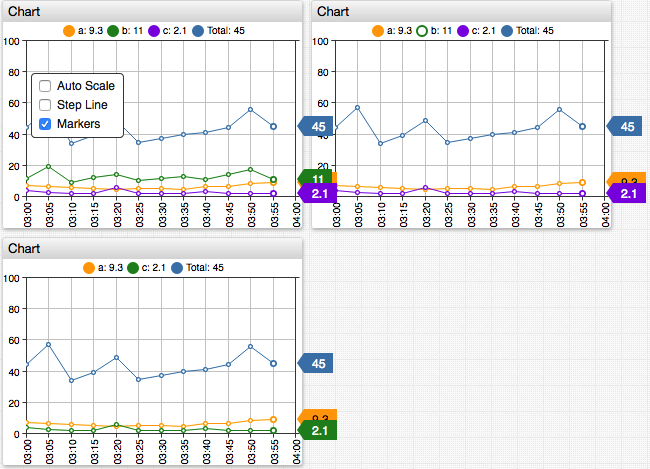# # Value Functions

## # Overview

This document describes fields and functions, which can be referenced in the `value` setting to create derived series.

The `value` setting is specified in the `[series]` and `threshold` sections.

``````# Define the original series, which values used in creating a derived (computed) series.
# The original series must exist in the database
[series]
metric = cpu_busy
entity = nurswgvml007

# Specify an alias
alias = s-1

# Optionally, hide the original series
display = false

# Define the derived series by specifying an expression in the `value` setting
[series]
label = My New Series

# Specify an expression called for each `time:value` sample in the original series
value = 2 * value("s-1")
``````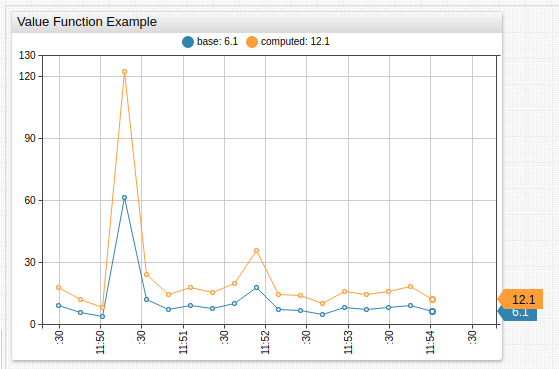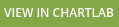The `value` expression is invoked for each `time:value` sample in the original series.

The expression must return a numeric value or `null` if the value cannot be calculated. `null` values are not displayed on the chart.

``````[series]
metric = cpu_busy
entity = nurswgvml007
alias = s-1
[series]
# Show values that exceed previous value by more than 10
value = value('s-1') > previous('s-1')+10 ? value('s-1') : null
label = s-2
``````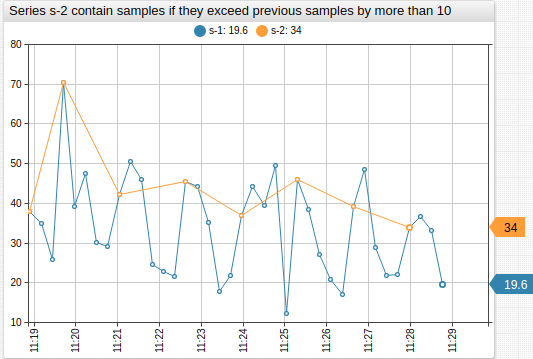The `time()` function can be invoked within the expression to check the timestamp of the current sample measured in Unix milliseconds.

``````value = var diff = value('s-2') - value('s-1'); return time() > new Date().getTime() ? null : diff;
``````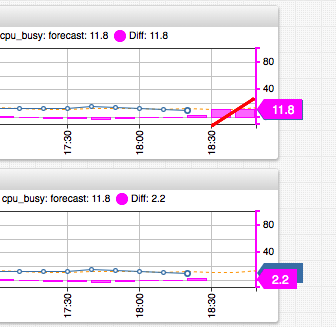Note

`value()` calculation is not affected by the visibility of the underlying series.

## # Lookup Functions

Function Arguments Description
`value` `alias` Value of the underlying series at the same timestamp.
`time` - Timestamp of the current sample in Unix milliseconds.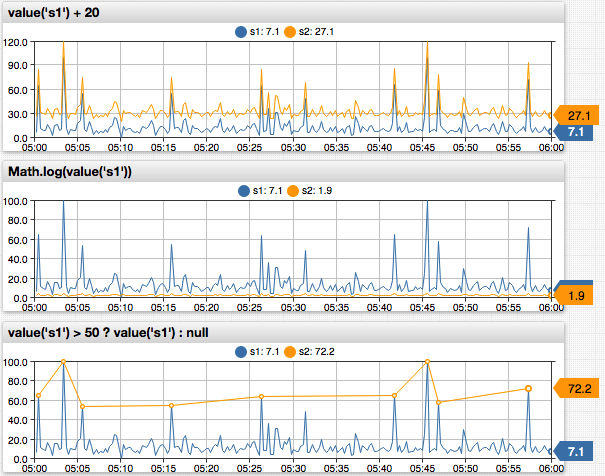## # Statistical Functions

• The `alias` argument is required. The `interval` argument is optional.
• If the interval argument is not specified, it depends on the `timespan` setting.
• If the timespan exceeds `interval`, series values are split into calendar-aligned periods and the function is applied to each period separately.
Function Arguments Description
`max` `alias`, `[interval]` Maximum within the specified interval.
`min` `alias`, `[interval]` Minimum within the specified interval.
`avg` `alias`, `[interval]` Average within the specified interval.
`sum` `alias`, `[interval]` Sum of values within the specified interval.
`delta` `alias`, `[interval]` Difference between last value in the specified interval and last value before the interval.
`counter` `alias`, `[interval]` Sum of positive differences between subsequent values within the specified interval.
`count` `alias`, `[interval]` Number of samples in the specified interval.
`last` `alias`, `[interval]` Value of the last sample in the specified interval.
`first` `alias`, `[interval]` Value of the first sample in the specified interval.
`min_value_time` `alias`, `[interval]` Timestamp of the maximum value in the specified interval.
`max_value_time` `alias`, `[interval]` Timestamp of the minimum value in the specified interval.
`median` `alias`, `[interval]` Same as `percentile(50)`.
`percentile` `n`, `alias`, `[interval]` `n`-th percentile, for example `percentile(75, 's1')` or `percentile(99.5, 's1')`.
`n` is a decimal number between `[0, 100]`.
`movavg` `alias`, `count`, `[minCount]` Returns average value of `count` last samples.
Returns `null` if the number of samples is less than `minCount`.
By default, `minCount` = `count`.
`movavg` `alias`, `interval`, `[minInterval]` Returns average value of last samples within `interval`.
Returns `null` if series contains less than one `minInterval` starting from current sample.
By default, `minInterval` = `interval`.
`previous` `alias`, `[offset]` Value of the previous sample, with optional `offset` index which defaults to `1`.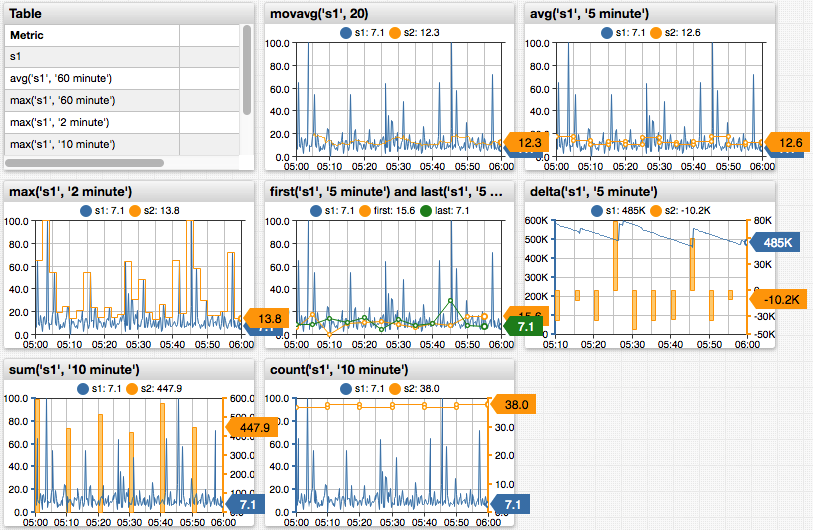Function Arguments Description
`meta` `alias` Metadata object.
`entityTag` `alias`, `tagName` Entity tag value.
`metricTag` `alias`, `tagName` Metric tag value.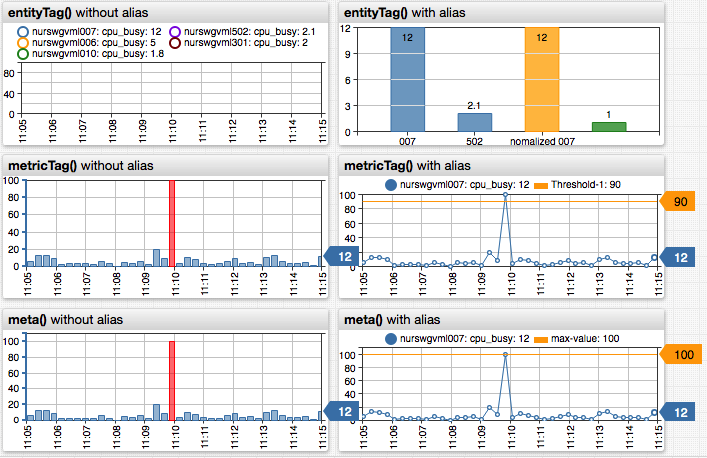## # Window Functions

Define a custom JavaScript function in the `window` object using the `script` / `endscript` section in the configuration text.

``````script
window.checkRange = function (val) {
if (val > 100) {
return null;
}
return val;
};
endscript
``````

The custom function can be accessed in the `value` field by referencing it by name.

``````value = return checkRange(value);
``````

Functions in the `window` scope can be invoked in other settings that support functions, for example, in the `format` setting.

## # Implementation Notes

### #`movavg(alias, count[, minCount])` Function

• Calculates the moving average using `count` previous points in the series defined by `alias`.
• The average is calculated if at least `minCount` previous points are available.
``````movavg(alias, count[, minCount])
``````
Name Type Description
`alias` string [Required] Alias of the series, to which `movavg` is applied.
`count` number [Required] Number of points for which `movavg` is calculated.
`minCount` number Minimum number of points, for which `movavg` is calculated, default is `count`.

Calculate `movavg` when a defined amount of points are available for calculation

``````value = movavg('raw', 30)
``````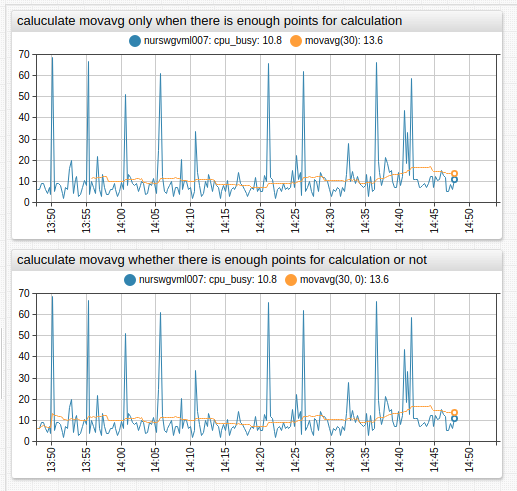Calculate `movavg` regardless of the number of points present

``````value = movavg('raw', 30, 0)
``````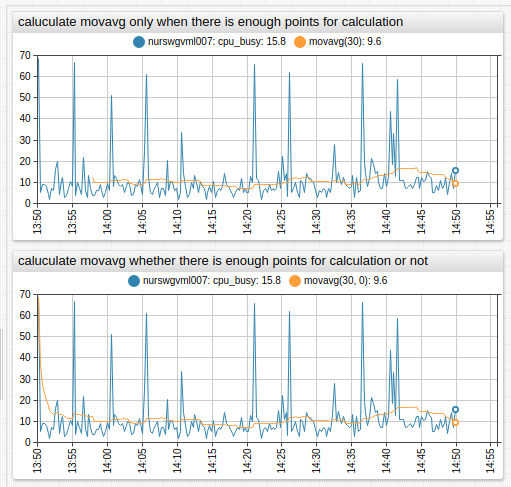### #`movavg(alias, interval[, minInterval])` Function

• Calculates the moving average using previous points within `interval` in the series defined by `alias`.
• The average is calculated if at least `minInterval` of previous points is available.
``````movavg(alias, interval[, minInterval])
``````
Name Type Description
`alias` string [Required] Alias of the series, to which `movavg` is applied.
`interval` interval [Required] Interval for which `movavg` is calculated, specified as the number of time units.
Format: `count time_unit`.
`minInterval` interval Minimum interval, for which `movavg` is calculated, default is `interval`.

Calculate `movavg` when a defined interval is available for calculation

``````value = movavg('raw', '5 minute')
``````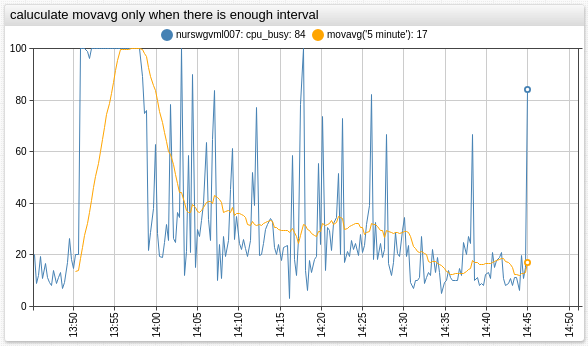Calculate `movavg` regardless of the defined interval present

``````value = movavg('raw', '5 minute', '0 minute')
``````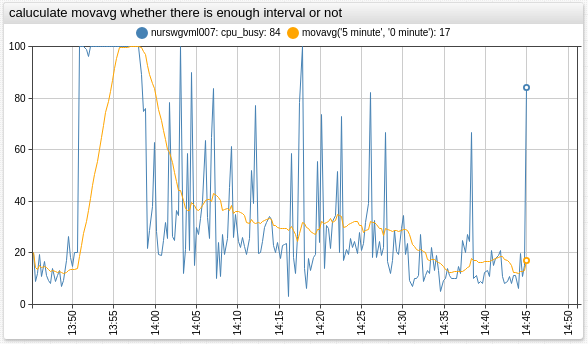### #`meta()` Function

``````meta(alias)
``````
Name Type Description
`alias` string [Required] Alias of the series, from which metadata is returned.

Fraction of `maxValue`

``````value = value('raw') / meta('raw').metric.maxValue
``````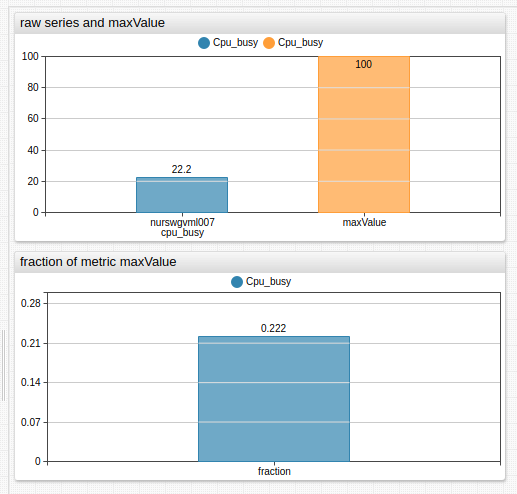### #`entityTag()` Function

• Returns value of the tag by name from entity metadata loaded for series with `alias`.
• `add-meta` setting must be set to `true`.
``````entityTag(alias, tagName)
``````
Name Type Description
`alias` string Alias of the series, from which metadata is returned.
`tagName` string Name of tag which is retrieved from `meta.entity.tags` object.

Set size to `cpu_count` entity tag

``````size = entityTag('cpu_count')
``````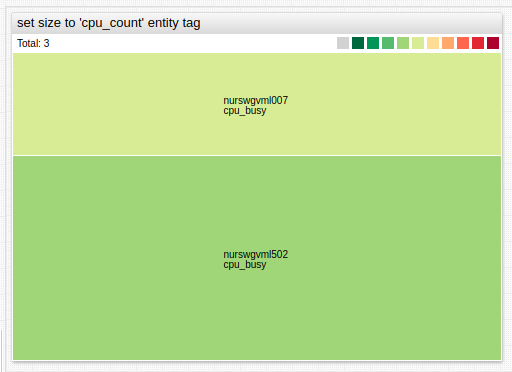### #`metricTag()` Function

• Returns value of the tag by name from metric metadata loaded for series with `alias`.
• `add-meta` setting must be set to `true`.
``````metricTag(alias, tagName)
``````
Name Type Description
`alias` string Alias of the series, from which metadata is returned.
`tagName` string Name of tag which is retrieved from `meta.metric.tags` object.

Set threshold to `threshold_value` metric tag

``````value = metricTag('raw', 'threshold_value')
``````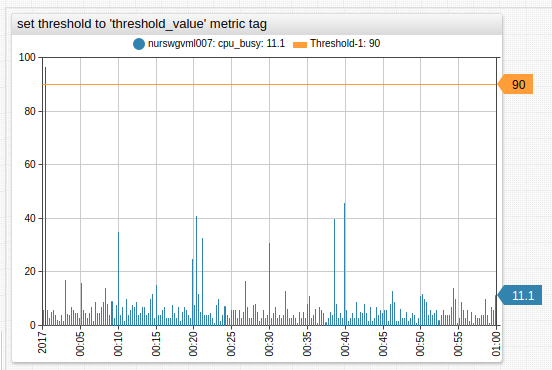### # Derived Series from Hidden Series

The `display` and `enabled` settings have no impact on the calculated series.

``````[series]
entity = nurswgvml006
alias = a

[series]
entity = nurswgvml007
alias = b
display = false

[series]
# The result is not affected by hiding the 'b' series
value = value('a') + value('b')
``````